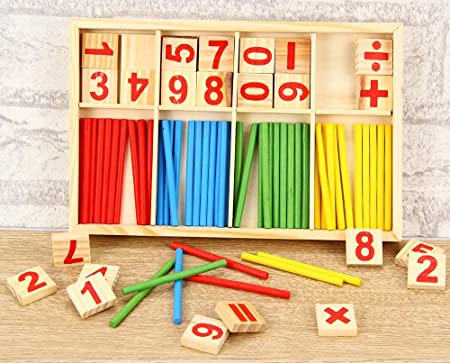# Amazing! Now Mathematics Gets Easier And Fun With These Models... Read On To Believe It!379The current approach to learning math in Singapore primary schools is through the math model method. Under this approach, students are required to draw math models that illustrate the questions. For lower primary students, modeling might seem unnecessary but the skills being mastered will come in handy at the upper primary level where problem sums becoming more challenging. To give your lower primary child a head start, this article will show you some techniques for mathematical modeling.

### 1. Background on math model method

This methodology was created by a Singaporean teacher called Hector Chee. Due to its practicality, the method was soon taught in all schools, starting from Primary One. This new method presented a challenge to parents who might have been taught using algebra or other math methods. As a result, many could not help their children to develop the right math model techniques for the different kinds of math problem sums.

### Main concepts in Singapore math model method

In the math model method, there are basically 2 concepts that form the foundation for all further iterations.

### The part-whole concept

In this concept, the child starts with understanding the relationship between parts. Once understood, they can represent these relationships using rectangular blocks to model math questions.

Let?s look at one example to better understand how the part-whole concept works. In the image below, the child starts off learning how to add the individual balls to understand a simple addition question of 3 +2 =5. At this stage, it is important to use real images such as the balls to let the child connect the dots.

### Singapore Math Model Method: Part-Whole Concept

Once the child has understood the above, we can take out the balls and uses blocks as representations. Below is how the image can be drawn. Once the kid accepts the blocks as representations, he or she will be in a good position to understand further abstraction.

### Singapore Math Model Method: Part-Whole Concept

After the above, we can go one step further to visualize the question in even more abstract terms. Here, we don?t need the individual blocks. Instead, just a visual distinction between 3 and 2 is enough to represent the relationship between the blocks.

### Singapore Math Model Method: Part-Whole Concept

In summary, this technique uses the relationship of the parts to let children learn about the whole.